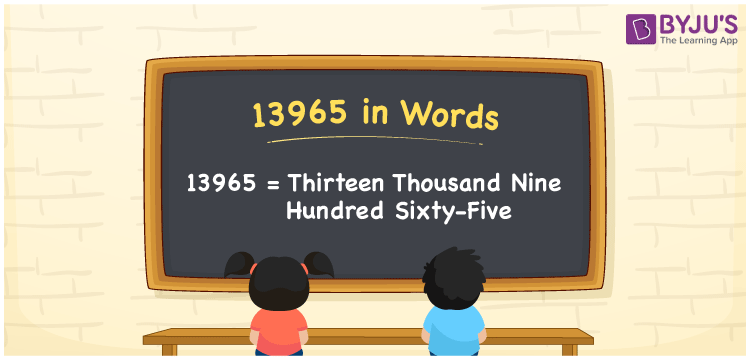# 13965 in Words

We can write 13965 in words as Thirteen thousand nine hundred sixty-five. If you purchased a mini coffee vending machine for Rs. 13965, then you can say, “I purchased a coffee vending machine worth Thirteen thousand nine hundred sixty-five rupees”. In this article, you will learn how to convert the cardinal number 13965 into words and some facts about 13965.

 13965 in words Thirteen thousand nine hundred sixty-five Thirteen thousand nine hundred sixty-five in Numbers 13965

## 13965 in English Words

Generally, we use the English alphabet to express numbers in words. So, we spell 13965 in English words as “Thirteen thousand nine hundred sixty-five”.## How to Write 13965 in Words?

Let’s learn how to write the number 13965 in words using a five-column place value chart given below.

 Ten thousand Thousands Hundreds Tens Ones 1 3 9 6 5

Here, ones = 5, tens = 6, hundreds = 9, thousands = 3, ten thousand = 1

The above digits can be expanded as follows.

1 × Ten thousand + 3 × Thousand + 9 × Hundred + 6 × Ten + 5 × One

= 1 × 10000 + 3 × 1000 + 9 × 100 + 6 × 10 + 5 × 1

= 10000 + 3000 + 900 + 60 + 5

= Ten Thousand + Three thousand + Nine hundred + Sixty + Five

= Thirteen thousand nine hundred sixty-five

Hence, 13965 in words = Thirteen thousand nine hundred sixty-five.

13965 is a natural number that is the successor of 13964 and predecessor of 13966.

13965 in words – Thirteen thousand nine hundred sixty-five

Is 13965 an odd number? – Yes

Is 13965 an even number? – No

Is 13965 a perfect square number? – No

Is 13965 a perfect cube number? – No

Is 13965 a prime number? – No

Is 13965 a composite number? – Yes

## Frequently Asked Questions on 13965 in Words

Q1

### How to write 13965 in words?

We can write 13965 in words as Thirteen thousand nine hundred sixty-five.
Q2

### How do you write an amount of Rs. 13965 in words?

The amount of Rs. 13965 can be written in words as “Thirteen thousand nine hundred sixty-five rupees”.
Q3

### What is 13965 in words?

13965 in words is expressed as Thirteen thousand nine hundred sixty-five, i.e., 13965 = Thirteen thousand nine hundred sixty-five.